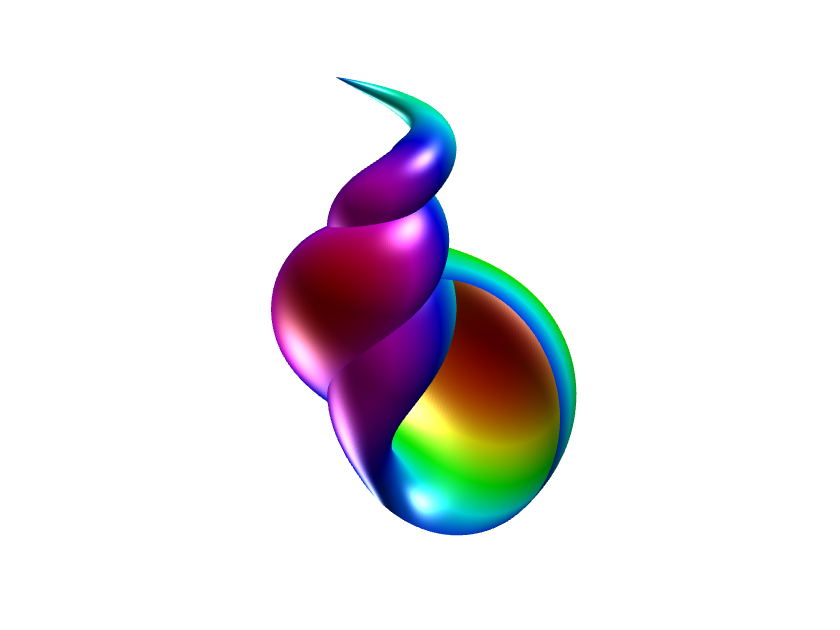# sinpi, cospi, implicit expansion and The 2022 MATLAB Mini-Hack

The 2022 MATLAB Mini-Hack is currently underway where MATLAB users submit 280 characters of code in order to produce a cool image. It's similar to last year's competition but this year you can also use functions from the Image processing toolbox and from File Exchange if you want to. An entry from Tim Davis, Professor of Computer Science at A&M University and author of SuiteSparse among many other things, caught the eye of a few of us earlier today. Here's the code
[u, v] = meshgrid (linspace (0, 2*pi, 512));
s = (1-v/(2*pi))/5;
t = .1 - s.*(1+cos(u));
x = t .* cos(2*v);
y = t .* sin(2*v);
z = v/(4*pi) - s.*sin(u);
surf (x ,y, z, y)
axis off equal
colormap (hsv (1024))
lightangle (80, -40)
lightangle (-90, 60)
view ([-150 10])A great result from just a few lines of code.
My fellow MathWorker, Bobby Cheng, made a remix of this that gave the same result using fewer characters by making use of three relatively new MATLAB capabilities: sinpi, cospi and implicit expansion. His remix can be seen at https://www.mathworks.com/matlabcentral/communitycontests/contests/5/entries/11588 and here is the code in full
u = linspace(0, 2, 512);
v = u.';
s = (1-v/2)/5 ;
t = .1 - s.*(1+cospi(u)) ;
x = t .* cospi(2*v) ;
y = t .* sinpi(2*v) ;
z = v/4 - s.*sinpi(u) ;
surf (x ,y, z, y)
axis off equal
colormap (hsv (1024))
lightangle (80, -40)
lightangle (-90, 60)
view ([-150 10])

## sinpi and cospi

The functions sinpi and cospi were released in MATLAB R2018b and, as well as helping us reduce character count in this code (which, although fun, isn't really all that useful!), they compute sin(x*pi) and cos(y*pi) without explicitly computing x*pi. Ultimately this is more accurate than doing the same thing with the traditional cos and sin functions because the floating point value of pi is an approximation of π. An obvious demonstration of this is
cos(pi/2)
ans = 6.1232e-17
cospi(1/2)
ans = 0

## Implicit expansion

Bobby removed the need for meshgrid by using implicit expansion, released in R2016b, and discussed in detail by Steve Eddins here and here.
Although not used in this code, implicit expansion can also remove the need for functions like ndgrid, repmat and bsxfun in many cases. For example, here's an example of how to get the same results as ndgrid using implict expansion
[x y] = ndgrid(1:3, 4:6);
x+y
ans = 3×3
5 6 7 6 7 8 7 8 9
x1 = (1:3).', y1 = 4:6
x1 = 3×1
1 2 3
y1 = 1×3
4 5 6
x1+y1
ans = 3×3
5 6 7 6 7 8 7 8 9

## The Bobby Cheng Challenge

Bobby made the assertion that many entries in the MATLAB Mini Hack could be simplified using the functions shown in this blog post. He challenged me to find some. Will you help me? Find an entry where the functionality mentioned in this blog post would reduce character count, create a remix and post the link to it as a comment to this blog post.
|